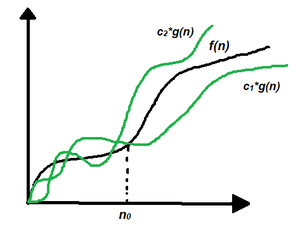# Analysis of Algorithms | Big – Θ (Big Theta) Notation

• Last Updated : 07 Aug, 2021

In the analysis of algorithms, asymptotic notations are used to evaluate the performance of an algorithm, in its best cases and worst cases. This article will discuss Big – Theta notations represented by a Greek letter (Θ).

Definition: Let g and f be the function from the set of natural numbers to itself. The function f is said to be Θ(g), if there are constants c1, c2 > 0 and a natural number n0 such that c1* g(n) ≤ f(n) ≤ c2 * g(n) for all n ≥ n0

Attention reader! Don’t stop learning now. Get hold of all the important DSA concepts with the DSA Self Paced Course at a student-friendly price and become industry ready.  To complete your preparation from learning a language to DS Algo and many more,  please refer Complete Interview Preparation Course.

In case you wish to attend live classes with experts, please refer DSA Live Classes for Working Professionals and Competitive Programming Live for Students.

Mathematical Representation:

Θ (g(n)) = {f(n): there exist positive constants c1, c2 and n0 such that 0 ≤ c1 * g(n) ≤ f(n) ≤ c2 * g(n) for all n ≥ n0}
Note: Θ(g) is a set

The above definition means, if f(n) is theta of g(n), then the value f(n) is always between c1 * g(n) and c2 * g(n) for large values of n (n ≥ n0). The definition of theta also requires that f(n) must be non-negative for values of n greater than n0

Graphical Representation:Graphical Representation

In simple language, Big – Theta(Θ) notation specifies asymptotic bounds (both upper and lower) for a function f(n) and provides the average time complexity of an algorithm.

Follow the steps below to find the average time complexity of any program:

1. Break the program into smaller segments.
2. Find all types and number of inputs and calculate the number of operations they take to be executed. Make sure that the input cases are equally distributed.
3. Find the sum of all the calculated values and divide the sum by the total number of inputs let say the function of n obtained is g(n) after removing all the constants, then in Θ notation its represented as Θ(g(n))

Example: Consider an example to find whether a key exists in an array or not using linear search. The idea is to traverse the array and check every element if it is equal to the key or not.

The pseudo-code is as follows:

bool linearSearch(int a[], int n, int key)
{
for (int i = 0; i < n; i++) {
if (a[i] == key)
return true;
}

return false;
}

Below is the implementation of the above approach:

## C++

 // C++ program for the above approach#include using namespace std; // Function to find whether a key exists in an// array or not using linear searchbool linearSearch(int a[], int n, int key){    // Traverse the given array, a[]    for (int i = 0; i < n; i++) {         // Check if a[i] is equal to key        if (a[i] == key)            return true;    }     return false;} // Driver Codeint main(){    // Given Input    int arr[] = { 2, 3, 4, 10, 40 };    int x = 10;    int n = sizeof(arr) / sizeof(arr);     // Function Call       if (linearSearch(arr, n, x))        cout << "Element is present in array";    else        cout << "Element is not present in array";     return 0;}

## Java

 // Java program for the above approachimport java.lang.*;import java.util.*; class GFG{ // Function to find whether a key exists in an// array or not using linear searchstatic boolean linearSearch(int a[], int n,                            int key){         // Traverse the given array, a[]    for(int i = 0; i < n; i++)    {                 // Check if a[i] is equal to key        if (a[i] == key)            return true;    }    return false;} // Driver codepublic static void main(String[] args){         // Given Input    int arr[] = { 2, 3, 4, 10, 40 };    int x = 10;    int n = arr.length;     // Function Call    if (linearSearch(arr, n, x))        System.out.println("Element is present in array");    else        System.out.println("Element is not present in array");}} // This code is contributed by avijitmondal1998

## Python3

 # Python3 program for the above approach # Function to find whether a key exists in an# array or not using linear searchdef linearSearch(a, n, key):     # Traverse the given array, a[]    for i in range(0, n):         # Check if a[i] is equal to key        if (a[i] == key):            return True         return False # Driver Code # Given Inputarr =  2, 3, 4, 10, 40x = 10n = len(arr) # Function Callif (linearSearch(arr, n, x)):    print("Element is present in array")else:    print("Element is not present in array")     # This code is contributed by shivanisinghss2110

## C#

 // C# program for above approachusing System; class GFG{ // Function to find whether a key exists in an// array or not using linear searchstatic bool linearSearch(int[] a, int n,                            int key){         // Traverse the given array, a[]    for(int i = 0; i < n; i++)    {                 // Check if a[i] is equal to key        if (a[i] == key)            return true;    }    return false;}  // Driver Codestatic void Main(){    // Given Input    int[] arr = { 2, 3, 4, 10, 40 };    int x = 10;    int n = arr.Length;     // Function Call    if (linearSearch(arr, n, x))        Console.Write("Element is present in array");    else        Console.Write("Element is not present in array");}} // This code is contributed by sanjoy_62.

## Javascript

 
Output
Element is present in array

In a linear search problem, let’s assume that all the cases are uniformly distributed (including the case when the key is absent in the array). So, sum all the cases (when the key is present at position 1, 2, 3, ……, n and not present, and divide the sum by n + 1.

Average case time complexity =⇒⇒⇒(constants are removed)

When to use Big – Θ notation: Big – Θ analyzes an algorithm with most precise accuracy since while calculating Big – Θ, a uniform distribution of different type and length of inputs are considered, it provides the average time complexity of an algorithm, which is most precise while analyzing, however in practice sometimes it becomes difficult to find the uniformly distributed set of inputs for an algorithm, in that case, Big-O notation is used which represent the asymptotic upper bound of a function f.

My Personal Notes arrow_drop_up